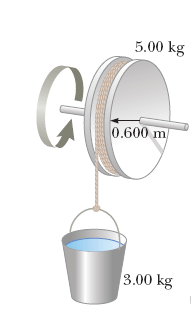# Problem: Use conservation of energy to determine the angular speed of the spool shown in Figure P8.52 after the 3.00-kg bucket has fallen 4.00 m, starting from rest. The light string attached to the bucket is wrapped around the spool and does not slip as it unwinds.

###### FREE Expert Solution

In this problem, we have gravitational potential energy, linear kinetic energy, and rotational kinetic energy.

Using conservation of energy:

$\overline{){{\mathbf{K}}}_{{\mathbf{0}}}{\mathbf{+}}{{\mathbf{U}}}_{{\mathbf{0}}}{\mathbf{+}}{{\mathbf{W}}}_{{\mathbf{nc}}}{\mathbf{=}}{{\mathbf{K}}}_{{\mathbf{f}}}{\mathbf{+}}{{\mathbf{U}}}_{{\mathbf{f}}}}$, where Wnc is the work done by non-conservative forces such as friction.

Linear kinetic energy:

$\overline{){\mathbf{K}}{\mathbf{=}}\frac{\mathbf{1}}{\mathbf{2}}{\mathbf{m}}{{\mathbf{v}}}^{{\mathbf{2}}}}$

Rotational kinetic energy:

$\overline{){\mathbf{K}}{\mathbf{=}}\frac{\mathbf{1}}{\mathbf{2}}{\mathbf{I}}{{\mathbf{\omega }}}^{{\mathbf{2}}}}$

Gravitational potential energy:

$\overline{){\mathbf{U}}{\mathbf{=}}{\mathbf{m}}{\mathbf{g}}{\mathbf{h}}}$

Moment of inertia of the spool:

$\overline{){\mathbf{I}}{\mathbf{=}}\frac{\mathbf{1}}{\mathbf{2}}{\mathbf{M}}{{\mathbf{R}}}^{{\mathbf{2}}}}$

90% (350 ratings)###### Problem Details

Use conservation of energy to determine the angular speed of the spool shown in Figure P8.52 after the 3.00-kg bucket has fallen 4.00 m, starting from rest. The light string attached to the bucket is wrapped around the spool and does not slip as it unwinds.